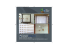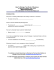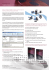# Credit Default Options with Mathematica 10

## Transcription

Credit Default Options with Mathematica 10
```Credit Default Options with Mathematica 10
Credit default options represent option products on the forward CDS rate and are credit analogy to the popular interest rate swap options. Due to this similarity,
they are also known in the market as CDS Swaptions. Swaption products developed to enable trading of credit default risk in the forward space where the
default protection might be required in the future. Being optional, CDS Swaptions represent the right, but not the obligation to enter into a CDS contract at predefined rate in the future. Since this right is valuable, the option holder pays the option premium. CDS Swaption pricing therefore determines how much the
holder needs to pay for such optional forward-starting insurance contract.
Similar to all previous cases, Mathematica 10 comes as ideal choice for this task as it offers rich probabilistic functionality, powerful integration and elegant
differentiation that are all required for this task.
To price credit default option, we need to know the forward
value of the CDS.
The value of forward CDS (FCDS) is trivial extension of the
standard CDS contract and we utilise all work done in the spot
space. Since we have defined the entire hazard rate / IR curve,
we can get the forward values of the hazard easily by the
1
[−ℎ(1)
following formula : ℎ = 2−1 [−ℎ{2} − 1
Although the CDS and FCDS share many
commonalities, they behave differently given the
time lag in the forward contract. The will generally
have a different shape:
1) CDS contract:
Consider a European call option on the CDS rate with
nominal =1 where we can buy protection on a
reference entity between times ,  for a fixed strike
(rate) . The value of this option is simply
0 = 0  [( − , 0] where 0 is the value of
the ‘risky annuity’ at time=0, covering the entire
product time span  is the risk-neutral expectation
with martingale setting and  is the forward value of
the FCDS.
And then specify both legs as:
Premium leg: =  ∗  ∗ ∑ [−( + ℎ) ∗ ]
Protection leg:= (1 − ) ∗  ∗ ℎ ∗ ∑ [−( + ℎ) ∗ ]
The meaning of all parameters is the same -  is the premium,
is the nominal amount,  is the risk-free zero –coupon rate,
ℎ is the forward hazard rate (default intensity),  is the
recovery rate and  is the year fraction – generally ¼.
Moving from spot-starting CDS to the forward CDS is simple in
Mathematica. One just needs to define different start and end
point in integration or summation in the discrete case:
There is one fundamental difference between IR and
credit swaption. Since the exercise of the option
means entry into a new IRS (CDS), in credit space this
will make sense only if the reference entity is still
‘active’, i.e. not defaulted. If the entity defaults during
the life of the option, the CDS swaption knocks out.
2) FCDS contract:
To value this option we need a model for  . A
standard assumption (analogy to the IR world) is to
assume that  is lognormal with zero drift and
volatility of ln  = √. This will generate a wellknown Black formula for the forward CDS rate:
We can use the Mathematica’s GBM process and get
the option value using the Expectation function of the
payoff formula:
Two lines of code produce complete analytical result
for this option. Numerical value can be obtained by
substitution.
Consider 3Y CDS swaption on 2Y CDS with the fixed strike
= 1.4% forward CDS rate 0 = 1.4% and the CDS volatility
= 28% using the curves defined earlier. The option
This is a typical representation of CDS path simulation
with the Gamma process:
Analytical solution is useful for a quick sensitivities
calculation:
1) Delta =
The IG model again produces elegant analytical call
option result:

0

2) Vega =
We can easily display the premium as a function of the FCDS
and its volatility
Other modelling assumptions for CDS Swaptions include
other Levy class – the so-called Inverse Gaussian model
[IG]. We parameterise the model to the same
underlying process:
To value an option with the Gamma process setting is
simple with Mathematica:
1) Define Gamma distribution
2) Parameterise it to the model:
a) Drift = 0 +
b) Volatility = √
3) Get the call option expectation with
martingale setting  = 0
The option premium is lower than Gamma, but higher
than LogNormal:
With Mathematica we can step into another level and
define even more complex model – say Gamma Process
with non-deterministic volatility . In our example we
assume the scale parameter b to follow exponential
process with parameter
Although this is quite complex setting, we still manage
to get a nice analytical result – in terms of Bessel and
Hypergeometric functions which Mathematica
evaluates easily:
With Mathematica we can go beyond the ‘standard’
Black swaption model. A suitable alternative is use the
so-called Levy stochastic processes which accounts for
jumps in the intensity regime (hazard rate). Gamma
process is well-represented Levy process suitable for the
credit risk modelling.
Gamma model generates surprisingly simple formula
and because of embedded jump dynamics, it results in
After substitution, we see even higher option premium.
Given the non-deterministic nature of the volatility,
this is reasonably expected:
In short: straightforward setting that make CDS
swaptions working just in few steps.
```

### 14 months cumulative work experience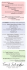### Editor`s Top Ten 2015 - Blues Music Magazine### New Release Chromeleon Goes MS – Next Steps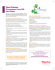### Nayelli Perez, M.S., CCDP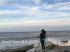### Through the acquisition of BQuotes, Moody`s offers comprehensive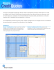### CDS - SeaBotix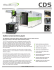### direct drive truckmounts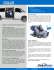### CinerArium Brochure - The UK`s Leading Cemetery Design